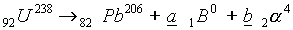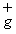SCHOLASTIC APTITUDE TEST 1997

CHEMISTRY

 Time: ONE Hour(12 Noon-1PM) Max.Marks : 60

• Instructions:

(1) Answer must be written either in English or the medium of instruction of the candidate in high school.

(2) There will be no negative marking

(3) There are THREE Sections. Answer all questions of a section at one place

(4) Use of calculators or graph papers is not permitted

SECTION-A

Explain the following Facts (write the equations wherever necessary) (15 x 2 = 30 Marks)

1. For the detection of CO2 lime water is used.

2. Electronegativity of N2 is considerable but it is used as inert gas.

3. For the detection of Nitrates in the laboratory, to the Nitrate and Ferrousulphate aqueous solution sulphuric acid is slowly added through the walls of the test tube.

4. PV = nRT (Gras equation) is not applicable to real gases under all conditions.

5. Graphite as well as diamond both are the allotropes of carbon but Graphite is used as lubricant, where as diamond is used as abrasive.

6. White phosphorus is always stored in water but sodium metal is stored in kerosene.

7. Bohr’s atomic model is superior than the Ruthurford atomic model.

8. Cl2 and SO2 both are bleaching agents, but only Cl2 is more frequently used as bleaching agent.

9. Across the period usually size of the atom decreases but size of the Inert gas in the given period is more than that of adjacent halogen.

10. Even though Zn is more reactive than Iron, surface of the Iron is converted with Zinc to protect from corrosion.

11. All minerals of a metal are not used for the extraction of metal.

12. Wooden shelf under the reagent bottle containing concentrated sulphuric acid blackens after some time.

13. Organic compounds don’t ionise when dissolved in water.

14. Electrolysis of Molten sodium chloride gives sodium and Cl2, but aqueous. Solution of NaCl gives Hydrogen and Cl2 gas.

15. Zinc displaces hydrogen from dilute acids but copper does not.

SECTION-B

Write the correct answers A,B,C,D,E: (5x11/2 = 71/2 Marks)

1. Three sets of quantum numbers are listed below pick the correct answer

 a) n = 2 l = 2 ml = 2 ms = 1/2 b) n = 4 l = 0 ml = 0 ms = 1/2 c) n = 3 l = 2 ml = 3 ms = 1/2 d) n = 3 l = 2 ml = 3 ms = 1/2
 A) a and b are allowed sets c is not B) b and c are allowed sets a is not C) all the 3 are allowed sets D) only b is an allowed set E) all 3 are not allowed sets

2. Given three statements below pick the correct answer

a) Cl has a higher electron affinity than P

b) the ionisation energy for Si is greater than pÅ

c) the electron affinity for `O’ is greater than the S

 A) a and c are true b is false B) b and c are true a is false C) a and b are true c is false D) all the true are true E) only b is true

3. Of the three which would be isoelectronic with oxygen atom

 a) N+ b) F- c) Ne2-
 A) a and b are isoeletronic c is not B) only a is isoelectronic C) only b is isoelectronic D) all are isoelectronic with oxygen E) none are isoelectronic with O

4. In the natural radioactive decay chain U238 undergoes a series of radioactive decays finally producing the stable nuclide Pb206.what are the values of a and b in this decay chain.

 A) a = 10, b = 16 B) a = 8, b = 6 C) a = 6, b = 8 D) a = 16, b = 10 E) none of these

5. Which of these statements are true?

A) if two salts have equal solubility their solubility products are equal

B) BaSO4 is more soluble in water than in dilute H2SO4

C) The solubility of a sparingly soluble salt is decreased by addition of a solute containing a common ion

D) If KI is added to a saturated solution of PbI2 the concentration of Pb2+ ions in the solution decreases

E) In a solution containing AgCl the product of concentration of Ag+ and Cl- is constant at constant temperature.

Match the Following (2 1/2 Marks)

 i). Aluminium hydroxide a. fine extinguisher ii). Ammonium sulphate b. refrigerant iii). Copper sulphate c. Amphoteric iv). Carbon dioxide d. Fertiliser v). Ammonia e. fungicide

SECTION-C

Fill in the Blanks: (20x1=20 Marks)

1. A large quantity of red phosphorus is used in match industry. The Matches which we use are called safety Matches. The head of the stick consists of _____________ and ____________ together with gum. The striking surface is composed of ___________, ____________ and fine glass to produce rough surface.

2. Pyrolusite is fused with KOH in the presence of air ____________ is formed.

3. 500 ml of 0.1 M HCl is treated with 250 ml of 0.1 Mole of NaOH. The amount of the heat liberated is ______________ k.cal.

4. Pressure remaining constant at what temperature the volume of a gas will be double of its volume at OoC __________________

5. The energy indicated in the equation Clg ® Cl+ e- - 1254 kJ is equal to ____________

6. Many thallous compounds are known but not thallic compounds because of _____________

7. The elements in which outer most shell incomplete are called _______________

8. As the temperature increases rate of reaction increases. This is due to ____________

9. Caffeine (Mol/wt = 194) contains 28.9% Nitrogen atoms in one Molecule of Caffeine is ___________

10. When 5p orbitals are filled completely, the differentiating electron enters into ______________

11. One mole of hydrogen iodide is heated in a closed container of capacity 2 lit. At equilibrium half a mole of hydrogen iodide has dissociated. The equilibrium constant is ______________

12. When zeolite which is hydrated sodium aluminium silicate is treated with hard water the sodium ions are exchanged with ________________ ions.

13. A Gaseous mixture contains oxygen and nitrogen in the ratio of 1:4 by weight. Therefore the ratio and their number of molecules is _____________

14. A mixture of sodium nitrate and ammonium chloride on strong heating gives rise to a colourless gas known as _______________

15. Radioactive isotope of hydrogen has ______________ neutrons.

16. Lithium is strongest reducing agent because of its ______________

17. A child has a temperature of 1040F . Body temperature of the boy in Celsius scale ___________

18. A supersaturated solution can form when temperature of a saturated solution is __________ and no crystals farm.

19. Major industrial source of hydrocarbons is ____________ which was formed from __________

20. Insufficient amounts of iodine in diet result in a condition called ___________, which is an __________ of the thyroid. This condition can be prevented by consuming Iodine containing foods such as _______________ and _______________